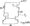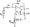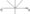# Linear IC’s and Applications July 2007

Note : 1. Answer any FIVE full questions,

2. Answers should be to the point,

3. Missing data, if any, may be assumed suitably.

1 a. Write a neat circuit diagram of a dual input balance output difference amplifier. Analyze the circuit using hybrid parameters and hence derive expressions for Ac, Ad and CMRR.

b. Calculate (Vi – V2) for the circuit shown: Assume identical silicon transistors with Vbe ~ 0.7 Volts. Amplitude of 3 V with a rise time of 4 fisec or less. Can a 741 be used?2 a. Explain why frequency compensation is necessary for an OPAMP. Discuss in detail different compensation methods.

b.  Compute the maximum possible total offset voltage in the circuit shown in fig.2(b) with the following specifications: Vj0 – 7.5 mV max, Ij0 ~ 50 nA max, Ib = 250 nA max at 25°C. 1) When Rcomp is used ii) Without RcompFig-2(b)

3 a.With a neat circuit diagram, obtain the expression for output voltage of a bridge amplifier. List some of its uses.

b.  Explain a symmetrical square wave generator using an OPAMP and hence derive an expression for its output frequency.

c.   List any four OPAMP parameters, which are very important in AC applications.

4 a. Write a suitable OPAMP circuit to obtain the transfer characteristics shown below in fig.4(a) and hence explain its working.Fig.4(a)

b. Design a 2nd order Butterworth low pass filter having high cutoff frequency of 1 kHz. Draw its frequency response.                                                                                 (10 Marks)

5 a. Explain a monostabie circuit using 555 timer and derive an expression for output pulse width.

b.  Explain the concept of fold back limiting in a 723 regulator IC.

6 a. Explain a 3 bit flash ADC with suitable circuit diagram.

b.  Calculate the values of LSB, MSB and full scale output for an 8 bit DAC for 0 to 10 V range.

c.   Design a voltage regulator using 723 to get a voltage output of 3 V.

7 a. Explain the application of PLL is frequency translation.

b.  List various analog to digital conversion techniques. Which is the faster ADC and why?

c.   Explain the principle of switching regulator.

8 Write short notes on the following:

a.   Instrumentation amplifier

b.  Three pin regulator

c.   V-I converter

d.  Averaging circuit.